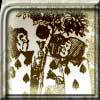#### You may also like### Painting Cubes

Imagine you have six different colours of paint. You paint a cube using a different colour for each of the six faces. How many different cubes can be painted using the same set of six colours?### Tri-colour

Six points are arranged in space so that no three are collinear. How many line segments can be formed by joining the points in pairs?### Cube Paths

Given a 2 by 2 by 2 skeletal cube with one route `down' the cube. How many routes are there from A to B?

# Permute It

##### Age 11 to 14 Challenge Level:

To add up all the permutations of all the 5 digit numbers written using the digits 1, 2, 3, 4 and 5 just once each time. A good way to tackle problems is to try simple cases and here you might try 21 + 12 = 33 then try it with 3 digits and so on. Ong Xing used the following method.

There are 5! numbers, and in each column of the sum each of the digits 1, 2, 3, 4 and 5 appears 4! = 24 times. The sum of each column is therefore 24(1 + 2 +3 + 4 +5) = 24 x 15 = 360.

The total sum is 360(1 + 10 + 102 + 103 + 104) = 360 x 11111 = 399960.

Joel used this even shorter argument. The number of permutations is 5!, that is 120. The average is 3, so the total is 33333 x 120 = 3999960.